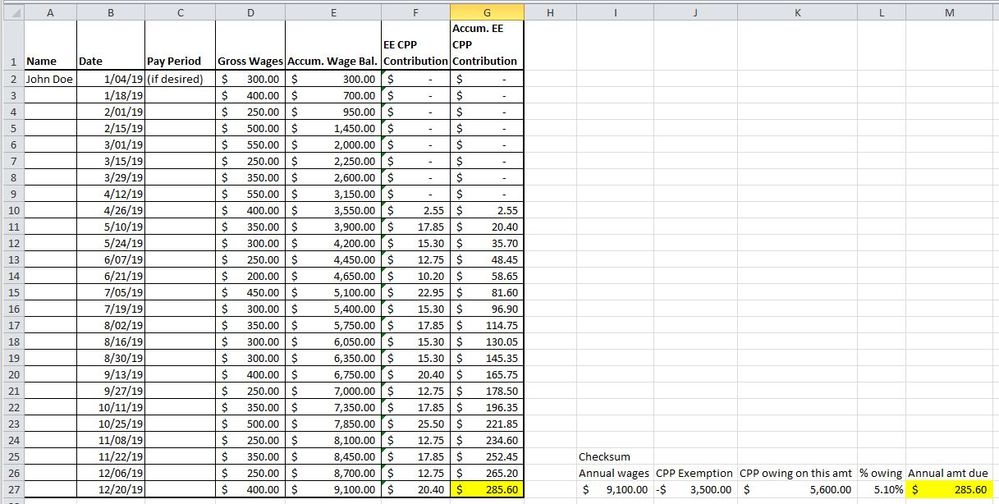# Employees and payroll

Do your part-time people make exactly the same wages each paycheque?  If so, make your own calculation by taking what they will make annually, deduct \$3,500 from annual wages and multiply by 5.1% = total CPP contributions owing by that Employee during the year.  Take the annual CPP amount and divide by remaining pay periods, then manually insert the result into each pay cheque in the Employee Summary until the end of the year.

If they don't make the same amount each pay, then you would need to create a spreadsheet to calculate the CPP owing, and manually enter Employee's CPP into the Employee Summary on each pay cheque.  I have attached a screenshot of someone working part time in 2019, and being paid bi-weekly.  From cell F2 to F10, the following formula was used:  =IF(E2>3500, E2*5.1%, 0), and copied down to the first cell where there is a result, in this case cell F10.  Beginning in cell F11, the formula is changed to:  =(E11-E10)*5.1%.  Column F are the amounts you would enter each pay period for the employee's CPP, at which time QB will automatically calculate the Employer's portion as normal.